# AP Calculus BC Practice Test 41

### Test Information10 questions20 minutes

Calculator Disallowed

1.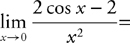2. If f(x) = x3 + 3x2 + cx + 4 has a horizontal tangent and a point of inflection at the same value of x, what is the value of c?

3.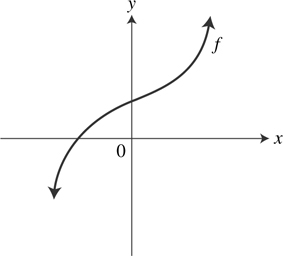The graph of f is shown above. Which of the following from above could be the graph of f′?

4. What are all values of x such that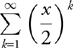converges?

5.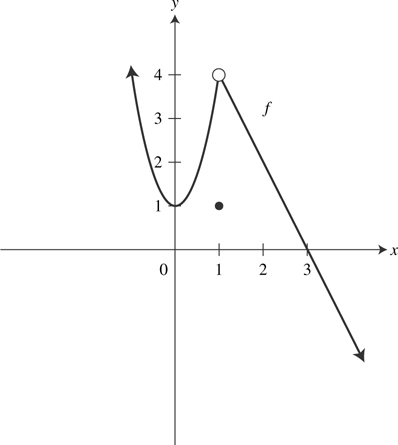The graph of a function f is shown above. Which of the following statements is/are true?

I.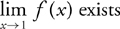II. f(1) exists

III.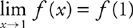6. What is the area of the region between the graph of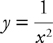and the x-axis for x ≥ 4?

7. If g(x) is continuous for all real values of x, then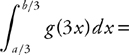8. .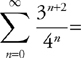9. The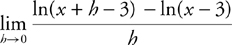is

10. Let f be a function with the following properties.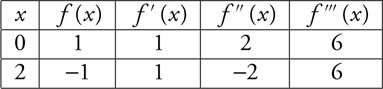Which of the following is the third-degree Taylor polynomial for f about x = 2?